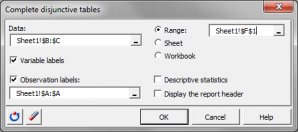# Complete disjunctive tables (Creating dummy variables)

Disjunctive tables are tables where columns are categories from one or several qualitative variables. Available in Excel with the XLSTAT statistical software.## What is a complete disjunctive table

A disjunctive table is a drill-down of a table defined by n observations and q qualitative variables V(1), V(2), ... V(q) into a table defined by n observations and p indicators (or dummy variables) where p is the sum of the numbers of categories of the q variables: each variable V(j) is broken down into a sub-table with q(j) columns where column k contains 1's for observations corresponding to the kth category and 0 for the other observations.

## How to create a complete disjunctive table with XLSTAT

Simply select a category variable in the XLSTAT tool for creating a complete disjunctive table. The new dummy variables are created within seconds.

Here is an example of complete disjunctive table. From a data table with two category variables, with respectively two and three modalities, a complete disjunctive table is created containing five dummy variables.

Data table:

 Q1 Q2 Obs1 A C Obs2 B D Obs3 B E Obs4 A D

Complete disjunctive table:

 Q1-A Q1-B Q2-C Q2-D Q2-E Obs1 1 0 1 0 0 Obs2 0 1 0 1 0 Obs3 0 1 0 0 1 Obs4 1 0 0 1 0### analyze your data with xlstat

14-day free trial

Included in

Related features